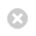Close

UCL Astrophysics Group

HomeThe Carina Nebula imaged by the VLT Survey Telescope Credit: ESO# A Statistical and Machine learning approach to Astrochemistry

Understanding the physical conditions in molecular gas and star-forming regions is an inverse problem subject to complicated chemistry that varies non-linearly with both time and the physical environment. Traditionally astrochemistry has always been dominated by trial and error grid-based analysis combined with simple statistics, an approach that becomes impossible or ineffective when datasets (e.g from ALMA) and/or parameter space are large, complex, or heterogeneous. It is time for a paradigm shift to be made in the way we interpret astrochemical models and fit them with the data, by using Bayesian as well as Machine Learning (ML) techniques.

Our group has already produced two proof of concept studies (Makrymallis & Viti 2014; Holdship et al. 2018), pioneering a new methodology in astrochemistry. In the first study we apply a Bayesian approach based on a Markov chain Monte Carlo (MCMC) method for solving the nonlinear inverse problems encountered in astrochemical modeling. We use observations for ice and gas species in dark molecular clouds and the time-dependent, gas-grain chemical model UCLCHEM to infer the values of the physical and chemical parameters that characterize quiescent regions of molecular clouds. In the second study we develop a simple model of grain surface chemistry in a molecular cloud and perform a Bayesian analysis through Markov Chain Monte Carlo sampling to infer the reactions rates (an example is shown in the Figure below). We are now in the process of generalize our algorithms to develop a method to infer reaction rates from observations of chemical abundances in astrophysical environments.Figure 1: The joint probability distributions of the rates of two reactions involving oxygen-bearing species. The darker areas represent higher probability densities. The 1,2 and 3 sigma contours are plotted. These two reactions are tightly coupled.

Credit: Prof Serena Viti